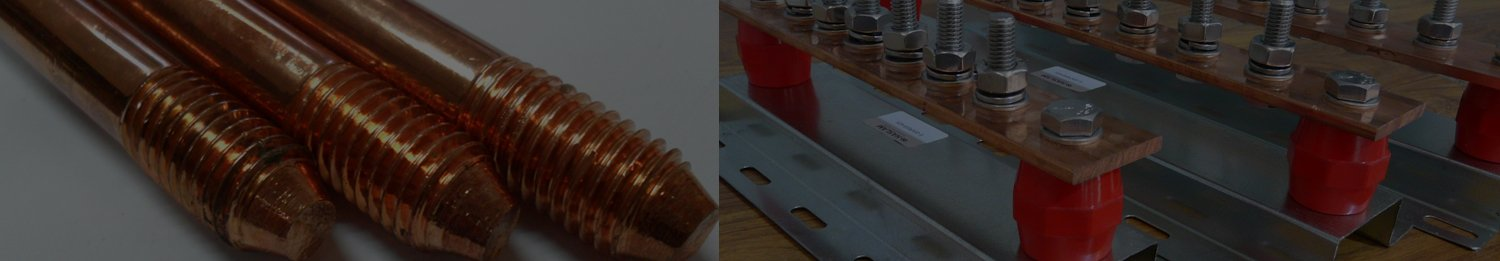# Copper Bar Weight Calculator

Results

To calculate the weight of a copper bar, you should know its dimensions (length, width, and thickness) and the density of copper. The formula to calculate the weight of an object is:

Weight = Volume × Density

For copper bars with a rectangular configuration, the volume can be ascertained by the formula:

Volume = Length × Width × Thickness

Copper, as a material, is known to possess a density of approximately 8.96 grams per cubic centimeter (g/cm³).

Incorporating all these elements into the calculation:

Weight (in grams) = Volume (in cm³) × Density (in g/cm³)
Weight (in grams) = (Length × Width × Thickness) × 8.96

Should the weight be desired in kilograms, a conversion can be achieved by dividing the weight in grams by 1000.

Remember that these principles are pivotal in accurately determining the weight of a copper bar while upholding precision and reliability in the calculation.